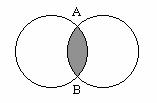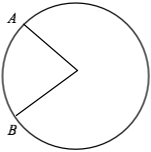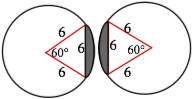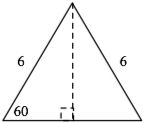## GMAT Strategy: Geometry Hybrids

One way the GMAT ramps up the difficulty of questions is to combine multiple concepts in a single problem. Geometry questions that do this can be challenging, particularly for test-takers who struggle to visualize alternative structures or orientations of a given figure.

Some of the most challenging of all Geometry Hybrids are those that fuse multiple figures into one larger figure and then ask about some facet of it. We call these Mixed Shapes, and categorize them into three groups: Overlapping Figures, Strange Shapes, and Shaded Areas. Let’s take a look at a sample problem to identify strategies to conquer these Geometry Hybrids.Two identical circles of area 36π overlap as shown above. If the distance from point A to point B is 6, what is the area of the shaded region?

(A)
(B) 12π
(C)– 6π
(D) 6π –(E) 12π –Before we get to the strategy, let’s review the three types of Mixed Shapes, and the tools to work them:

• Overlapping Figures – usually combine two common figures that share the same space. To work them, try to find the shared measure.
• Strange Shapes – these usually fuse two or more common shapes into a larger, uncommon or irregular shape. To work them, try to cut the irregular shape into easy-to-manage common shapes.
• Shaded Areas – these usually shade a part of one or more figures. To work them, add or subject the unshaded area of the figure from the total area of the figure.

With that out of the way, here’s the strategy for these problems:

1. Identify the basic shapes and properties of the figures involved.

2. Identify which Mixed Shapes concepts are being tested.

3. Apply strategies for each concept to the information given.

4. Solve.

Let’s apply these steps to the problem above.

1. This question involves circles. Important circle information includes Area, Circumference, and Radius. Since the figures given are circles, it is logical to draw radii to the edges of the shaded region.Drawing these radii reveals that we have a of a circle. Since we are given the length of AB draw that in and label it. Since the area of the circle is 36π the must be 6 and the created must be .

2. The two mixed shape concepts involved here are shaded areas and overlapping figures, which means we’re looking to find the unshaded quantities to add or subtract them to find the unknown shaded area. This also means we’ll be looking for a shared measure, which in this case would be the chord that runs down the middle of the shaded area from A to B.

3. Apply the information:

Drawing the two circles separately and labeling all information would look like this:Now we can see that we have manageable shapes (Triangles and Sectors of a Circle) with which to calculate the shaded areas.

4) Solve.

Area of the triangle:Dropping the height reveals a 30 – 60 – 90 (which has sides x, x√3, and 2x respectively).

Since the 2x side must be across from the 90° degree angle, 2x = 6 and x = 3. Therefore the height is 3√3 and the base is 6 (the side of the full triangle). The area is 9√3.

Area of the sector (from the Arc-Sector Formula):

60/360 = x/36π

x = 6π

Thus, the area of the shaded region is the difference between the area of the circle sector and the area of the triangle. The area of the shaded region in one of the circles is 6π– 9√3. The area of the shaded region in both circles would be twice that , or 12π– 18√3.  Consequently, the answer is (E).

It is important to realize that to solve these kinds of questions, we need to deconstruct them to find their more manageable component parts, then understand the relationship between the different parts, then apply our knowledge, and then solve. Trying to solve without first understanding what you’re working with will make these problems more difficult to solve and more time consuming.

Good luck, and happy Geo-hybriding!

-The Bell Curves Team

• (646) 414-1586
CONNECT WITH US